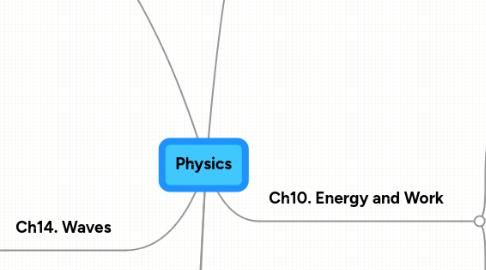# Physics

Get Started. It's FreePhysics## 1. Ch27. Quantum Energy

### 1.1. 27.1 A Particle model of Waves

1.1.1. Key concepts

1.1.1.1. Objects that are hot enough to be incandescent emit lightbecause of the vibrations of the charged particles inside their atoms.

1.1.1.2. The spectrum of incandescent objects covers a broad range of wavelengths. The spectrum depends upon the temprature of the incandescent objects.

1.1.1.3. Planck explained that spectrum of an incandescent object by soppusing that a particle can have only certain energies that are multiples of a constant, now called Planck's constant.

1.1.1.4. Einstien explained the photoelectric effect by postulating that light exists in bundles of energy called photons.

1.1.1.5. The photoelectric effect is the emission of electrons by certain metals when they exposed to electromagnetic radiation

1.1.1.6. The photoelectric effect allows the measurement of Planck's constant, h

1.1.1.7. The work function, which is equivelant to the binding energy of the electron, is measured by the threshold frequency in the photoelectric effect.

1.1.1.8. The Compton effect demonstrates that photons have momentum, as predicted by Einstien.

1.1.1.9. Even though photons, which travel at the speed of light, have zero mass, they do have energy and momentum.

1.1.2. Formulas

1.1.2.1. E=nhf

1.1.2.2. E=hf=hc/>=1240eVxnm/>

1.1.2.3. KE=hf-hf*

1.1.2.4. p=fc/c=h/>

### 1.2. 27.2 Matter Waves

1.2.1. Key concepts

1.2.1.1. The particle and wave aspects are the complementary parts of the complete nature of both matter and light.

1.2.1.2. The Heisenburg uncertainty principle states that it is not possible to simultaneously measure the precise position and momentum of any light particle

1.2.2. Formulas

1.2.2.1. >=h/p=h/mv

## 2. Ch14. Waves

### 2.1. 14.1 Periodic Motion

2.1.1. Key concepts

2.1.1.1. Periodic motion is any motion that repeats in a regular cycle

2.1.1.2. Simp Harmonic motion results when the restoring force on an object is directly propertional to the objects displacement from equilibrium. Such a Force Obeys Hooke's law.

2.1.1.3. The Elastic potential energy stored in a spring obeys that obeys Hooke's law is expressed by the equation PE=1/2kx*2

2.1.2. Formulas

2.1.2.1. F=-kx

### 2.2. 14.2 Wave Properties

2.2.1. Formulas

2.2.1.1. Waves transfer energy without transferring matter

2.2.1.2. In Transverse waves, the displacement of the medium is perpendicular to the direction of the wave motion. In longitudonal waves, the displacement is parallel to the wave motion.

2.2.1.3. Frequency is the number of cyvles per second.

### 2.3. 14.3 Wave Behavior

2.3.1. Key concepts

2.3.1.1. Wave length= v/f

2.3.2. Formulas

2.3.2.1. When a wave crosses a boundry between two media, it is partially transmitted and partially reflected

2.3.2.2. The principle of superposition states that the displacement of a medium resulting from two or more waves is the algebraic sum of the displacements of the individual waves.

2.3.2.3. Interference occurs when two or more waves move through a medium at the same time.

2.3.2.4. When two-dimensional waves are reflected from boundries, the angles of incidence and reflection are equal.

2.3.2.5. The change in direction of waves at the boundry between two diffrent media is called refraction

## 5. Ch11. Conservation of Energy

### 5.1. 11.2 Conservation of Energy

5.1.1. Key concepts

5.1.1.1. The Kinetic energy of an object is proportional to its mass and the square of its velocity

5.1.1.2. The rational kinetic energy of an object is proportional to the objects moment of inertia and the square of its angular velocity

5.1.1.3. When Earth is included in a system, the work done by gravity is replaced by gravitational potential energy

5.1.1.4. The gravitational potential energy of an object depends on the objects weight and its distance from earths surface

5.1.1.5. The reference level is the position where the gravitational potential energy is defined to be zero

5.1.1.6. Elastic Potential Energy may be stored in an object as a result of its change in shape

5.1.1.7. Albert Einstien recognised that mass itself has potential energy. This energy is called rest energy

5.1.2. Formulas

5.1.2.1. PE=mgh

5.1.2.2. E=mc*2

### 5.2. 11.1 The many forms of Energy

5.2.1. Key concepts

5.2.1.1. The sum of kinetic potential energy is called mechanical energy

5.2.1.2. If no objects enter or leave a system, the system is considered to be a closed system

5.2.1.3. If there are no external forces acting on a system, the system is considered to be an isolated system

5.2.1.4. The energy of a closed, isolated system is constant. Within the system, energy can change form, but total energy does not change, thus the energy is conserved.

5.2.1.5. The type of collision in which the kinetic energy after the collision is less than the kinetic energy before the collision is called an inelastic collision

5.2.1.6. The type of collision in which the kinetic energy before and after the collision is the same is called an elastic collision

5.2.1.7. Momentum is conserved in collisions if the external force is zero. The mechanical energy may be unchanged or decreased by the collision, depending on whether the collision is elastic or inelastic

5.2.2. Formulas

5.2.2.1. E= KE+PE

5.2.2.2. KE(b4) + PE(b4) = KE + PE After

## 6. Ch10. Energy and Work

### 6.1. Key Concepts

6.1.1. Work is the transfer of energy by mechanical means.

6.1.2. A moving object has Kinetic Energy

6.1.3. The Work done on a system is equal to the change in energy of the system

6.1.4. Work is the product of the force exerted on an object and the distance the object moves in the direction of the force

6.1.5. The work done can be determined by calculating the area under a force-displacement graph

6.1.6. Power is the rate of doing work, that is the rate at which energy is transmitted

### 6.2. Formulas

6.2.1. W=Fd

6.2.2. KE=1/2 mv2

6.2.3. W=(change)KE

6.2.4. W=Fd cos teta

6.2.5. P= W/t